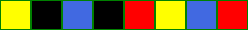Proposed by Toby Walsh

Consider two sets of the numbers from 1 to 4. The problem is to arrange the eight numbers in the two sets into a single sequence in which the two 1’s appear one number apart, the two 2’s appear two numbers apart, the two 3’s appear three numbers apart, and the two 4’s appear four numbers apart.

The problem generalizes to the L($k$,$n$) problem, which is to arrange $k$ sets of numbers 1 to $n$, so that each appearance of the number $m$ is $m$ numbers on from the last. For example, the L(3,9) problem is to arrange 3 sets of the numbers 1 to 9 so that the first two 1’s and the second two 1’s appear one number apart, the first two 2’s and the second two 2’s appear two numbers apart, etc.

A graphical representation of L(2,4), with black=1, red=2, blue=3 and yellow=4 is given below.